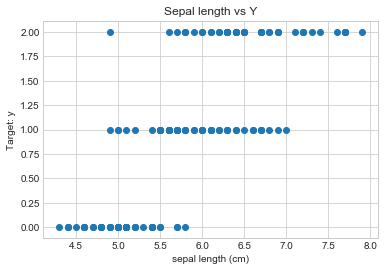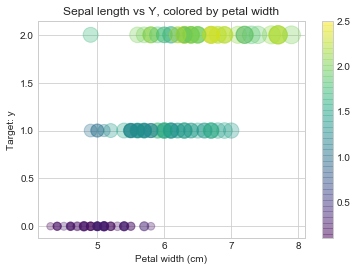# Scatterplot Chart¶

#### Some preliminaries¶

If you are wondering why in the world this webpage looks the way it does, it might help you to review Anaconda, Jupyter scripts and a basic Python example. You can do so by reviewing the post(s) below.

### Data Exploration with Scatterplots¶

We start our foray into scatterplots using the popular Matplotlib library (https://matplotlib.org/). This was inspired from MATLAB, and provides a lot of control over nearly every aspect of the chart (at the cost of lots of coding!).

We load in a popular predictive modeling dataset called "Iris" using the sklearn library. Then, we utilize scatter plots to visualize the data and its relationship with the variable that we are trying to predict.

In :
import pandas as pd
data = pd.DataFrame(iris.data, columns=[iris.feature_names]) #loading data into a pandas dataframe (for easier manipulation)

In :
data['y'] = pd.Series(data=iris.target, index=data.index) #the loaded data does not include the target variable for some reason, adding it here
data.describe() #get some basic stats on the dataset

Out:
sepal length (cm) sepal width (cm) petal length (cm) petal width (cm) y
count 150.000000 150.000000 150.000000 150.000000 150.000000
mean 5.843333 3.057333 3.758000 1.199333 1.000000
std 0.828066 0.435866 1.765298 0.762238 0.819232
min 4.300000 2.000000 1.000000 0.100000 0.000000
25% 5.100000 2.800000 1.600000 0.300000 0.000000
50% 5.800000 3.000000 4.350000 1.300000 1.000000
75% 6.400000 3.300000 5.100000 1.800000 2.000000
max 7.900000 4.400000 6.900000 2.500000 2.000000
In :
data.head() #we observe the first few lines of the dataset (always a good idea to get a sense of what is "in there")

Out:
sepal length (cm) sepal width (cm) petal length (cm) petal width (cm) y
0 5.1 3.5 1.4 0.2 0
1 4.9 3.0 1.4 0.2 0
2 4.7 3.2 1.3 0.2 0
3 4.6 3.1 1.5 0.2 0
4 5.0 3.6 1.4 0.2 0
In :
import matplotlib.pyplot as plt
x = data[["sepal length (cm)"]] #our 'x' axis variable
y = data[["y"]] #our target or 'y' axis variable. In the iris dataset this is the classification (0=
plt.style.use('seaborn-whitegrid') #experiment with different options for the look/feel of chart: 'seaborn-darkgrid', 'ticks', 'white', 'dark'
# Notes: 'alpha' controls transparency of the markers.
plt.scatter(x, y) #you might have to run this cell twice for the plot to show
plt.title("Sepal length vs Y")
plt.xlabel("sepal length (cm)")
plt.ylabel("Target: y")
plt.show()In :
colors = data[["petal width (cm)"]] #display another dimension in our plot by using another variable that controls 'color' of markers
sizes = 50*data[["petal length (cm)"]] #we can use another dimension in our plot by using another variable that controls 'size' of markers. Note the multiplication factor, which you can set by hit/trial to see what looks like the right size.
# Notes: 'alpha' controls transparency of the markers.
plt.scatter(x, y, c=colors, s=sizes, marker='o', alpha=0.3,
cmap='viridis') #you might have to run this cell twice for the plot to show
plt.title("Sepal length vs Y, colored by petal width")
plt.xlabel("Petal width (cm)")
plt.ylabel("Target: y")
plt.colorbar() #show the colorbar
plt.show()You can use scatterplots to visualize the relationship of variables. For example, in the chart above we can see that lower petal width corresponds to target variable designated as '0' and higher corresponds to '1' or '2'.

Helpful tip: you can use the 'snipping tool' in windows to cut/paste any developed charts into your report, presentation, or to save it as a picture and use whereever you like.